# Ratios Rates and Unit Rates across the Universe

• Slides: 14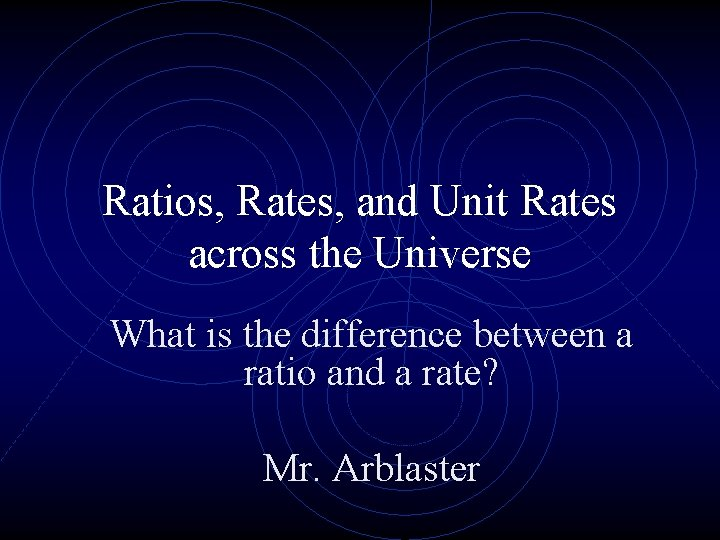Ratios, Rates, and Unit Rates across the Universe What is the difference between a ratio and a rate? Mr. Arblaster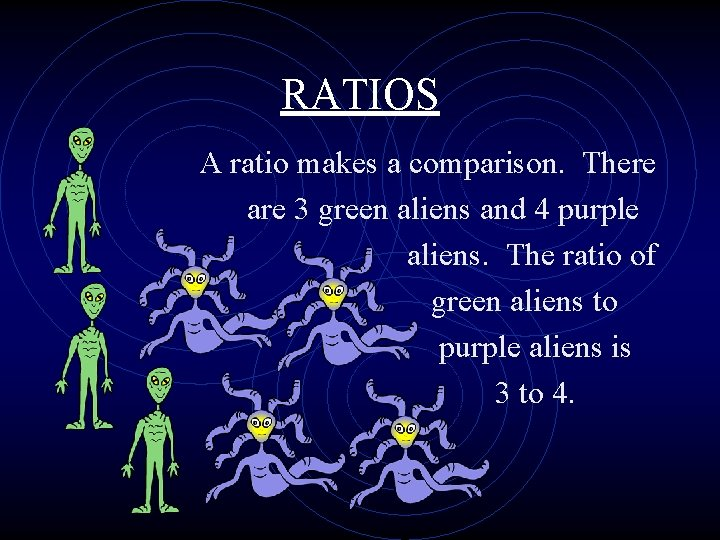RATIOS A ratio makes a comparison. There are 3 green aliens and 4 purple aliens. The ratio of green aliens to purple aliens is 3 to 4.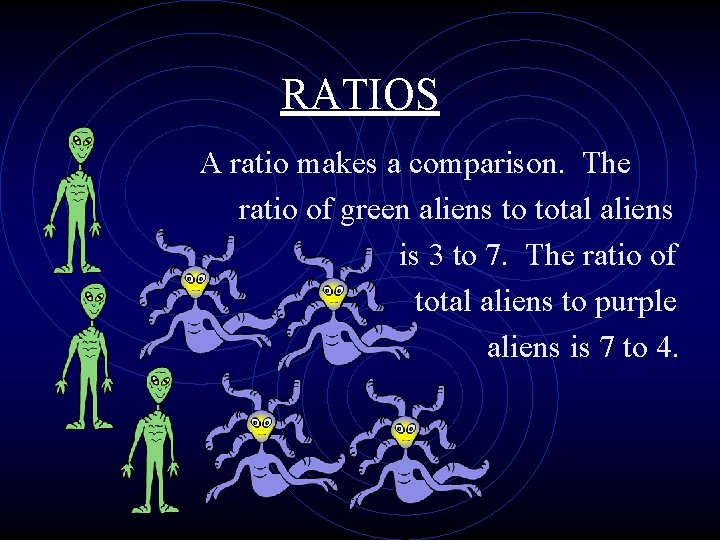RATIOS A ratio makes a comparison. The ratio of green aliens to total aliens is 3 to 7. The ratio of total aliens to purple aliens is 7 to 4.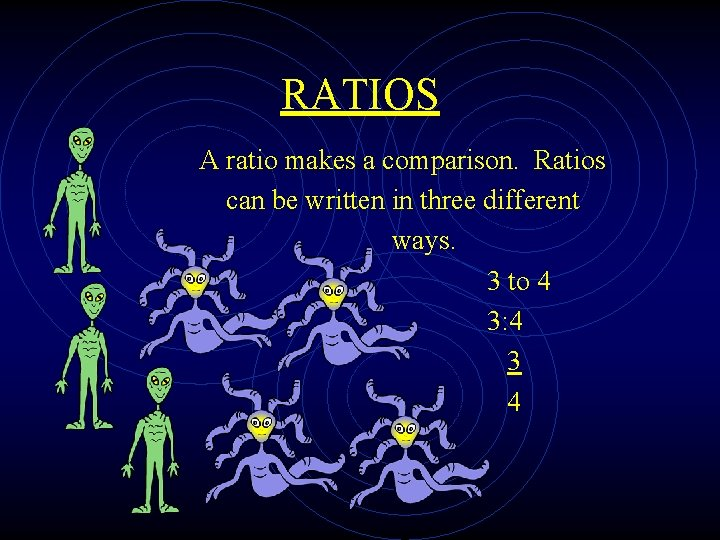RATIOS A ratio makes a comparison. Ratios can be written in three different ways. 3 to 4 3: 4 3 4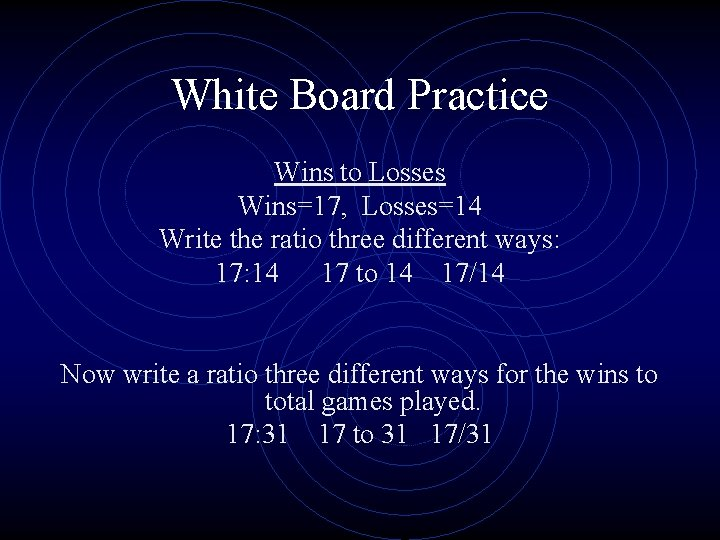White Board Practice Wins to Losses Wins=17, Losses=14 Write the ratio three different ways: 17: 14 17 to 14 17/14 Now write a ratio three different ways for the wins to total games played. 17: 31 17 to 31 17/31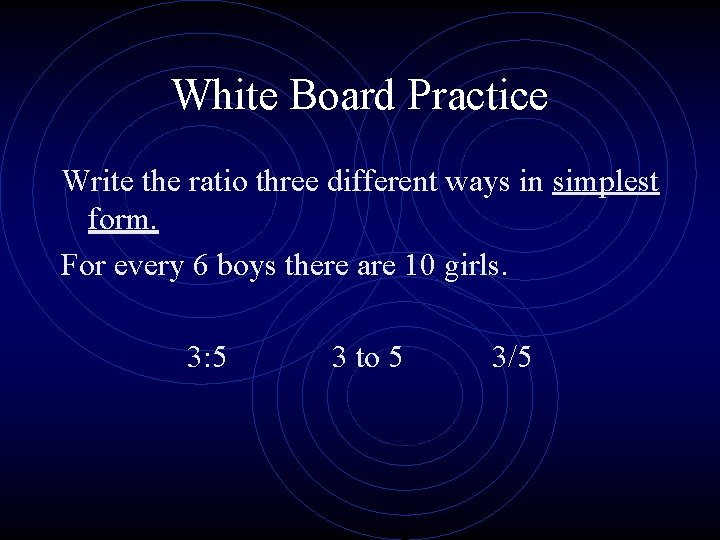White Board Practice Write the ratio three different ways in simplest form. For every 6 boys there are 10 girls. 3: 5 3 to 5 3/5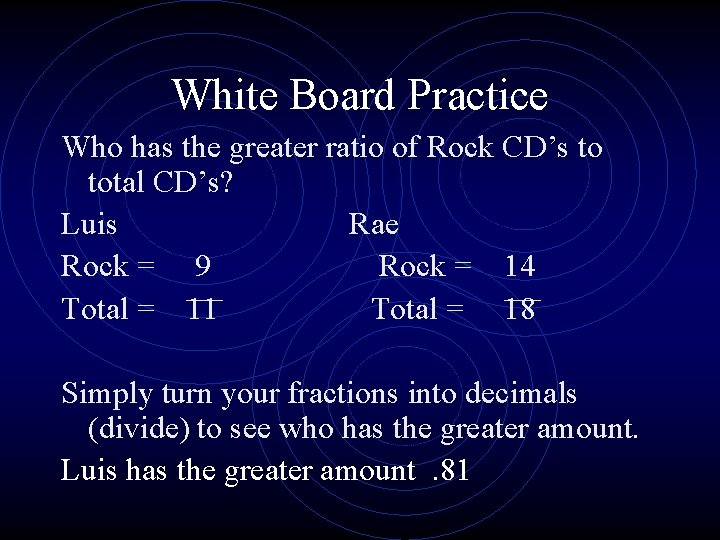White Board Practice Who has the greater ratio of Rock CD’s to total CD’s? Luis Rae Rock = 9 Rock = 14 Total = 11 Total = 18 Simply turn your fractions into decimals (divide) to see who has the greater amount. Luis has the greater amount. 81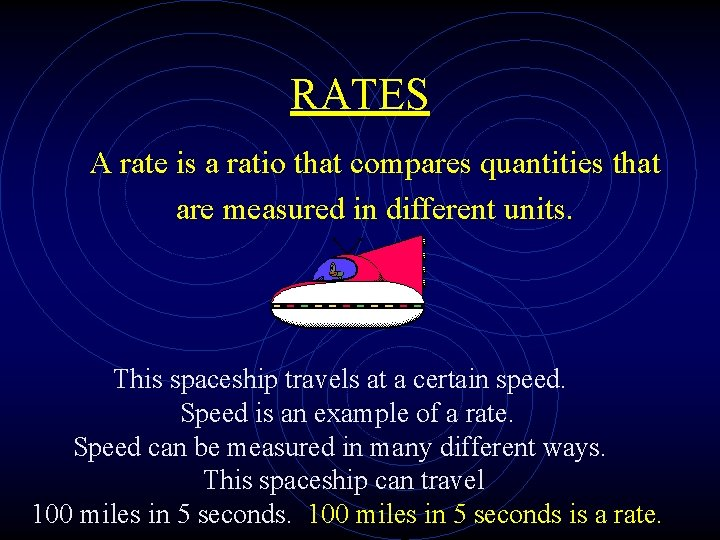RATES A rate is a ratio that compares quantities that are measured in different units. This spaceship travels at a certain speed. Speed is an example of a rate. Speed can be measured in many different ways. This spaceship can travel 100 miles in 5 seconds is a rate.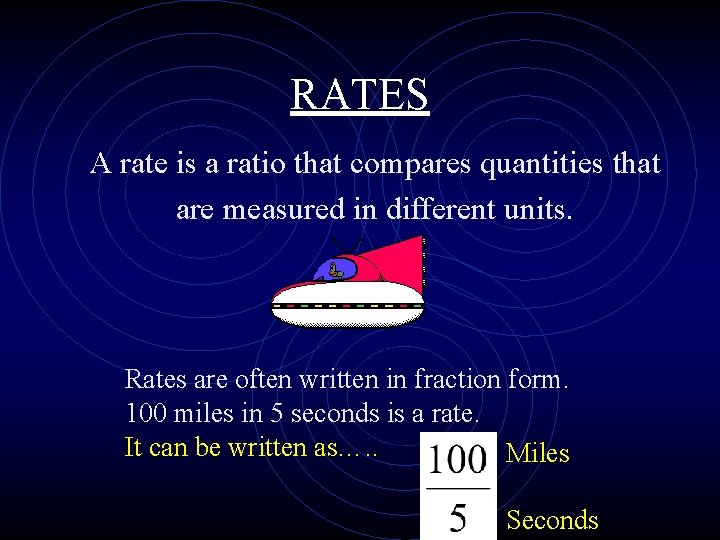RATES A rate is a ratio that compares quantities that are measured in different units. Rates are often written in fraction form. 100 miles in 5 seconds is a rate. It can be written as…. . Miles SecondsRATES A rate is a ratio that compares quantities that are measured in different units. One key word that often identifies a rate is PER. Miles per gallon, Points per free throw, Dollars per pizza, Sticks of gum per pack What other examples of rates can your group think of?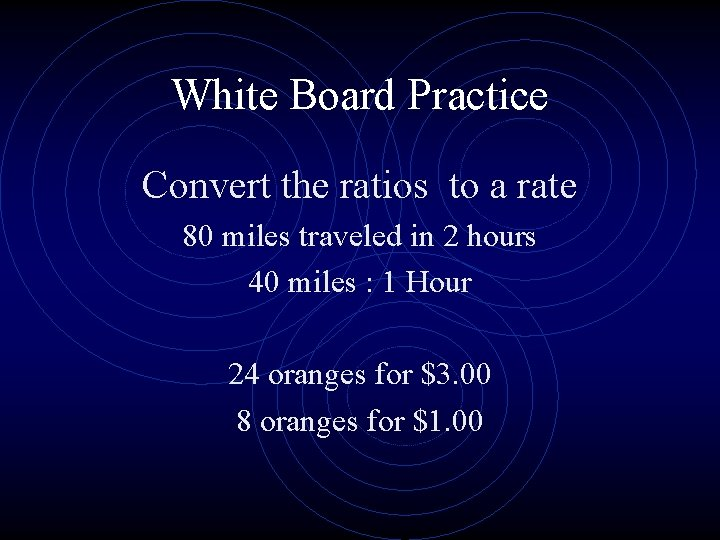White Board Practice Convert the ratios to a rate 80 miles traveled in 2 hours 40 miles : 1 Hour 24 oranges for \$3. 00 8 oranges for \$1. 00UNIT RATES Most of the time when we work with rates we use a unit rate. A unit rate is a ratio that compares a value to 1 unit. Unit Rates ALWAYS have a 1 for a denominator. This alien can walk at a rate of 10 miles in 2 hours. His speed is a unit rate of 5 miles per 1 hour or simply 5 miles per hour. RATIO RATE Miles Hours Miles = Hour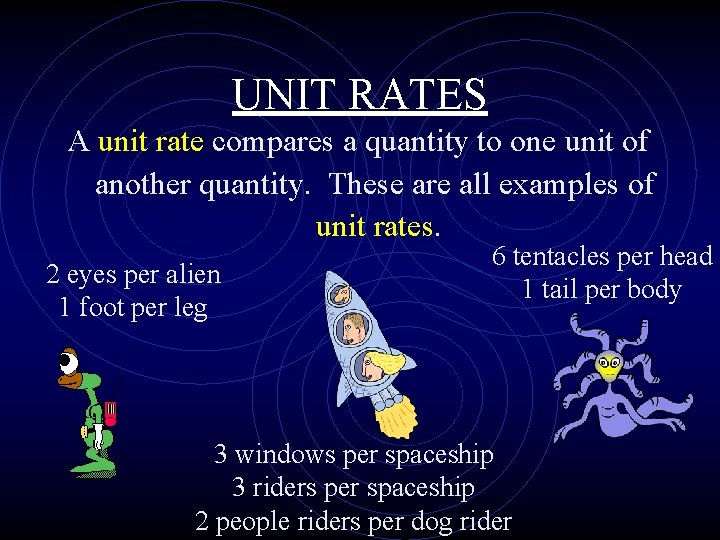UNIT RATES A unit rate compares a quantity to one unit of another quantity. These are all examples of unit rates. 2 eyes per alien 1 foot per leg 6 tentacles per head 1 tail per body 3 windows per spaceship 3 riders per spaceship 2 people riders per dog rider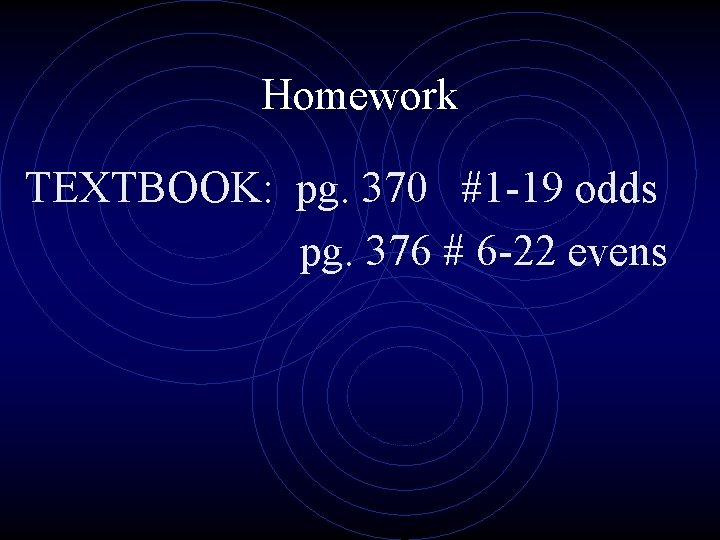Homework TEXTBOOK: pg. 370 #1 -19 odds pg. 376 # 6 -22 evens